[Next] [Up] [Previous] [Index]

# Factoring

Because d can be derived from e once (p-1)(q-1) is known, factoring M into its prime factors p and q is a way of cracking the RSA cipher.

Factoring large numbers is difficult. There are ways to improve on dividing a number by every prime number that is smaller than it is. The first is to note that one only needs to divide by the numbers smaller than the square root of the number to be factored. But other improvements are possible.

One relatively easy-to-understand improved algorithm for factoring is called Fermat factorization. This makes use of the fact that

```               2  2
(a+b)*(a-b) = a -b
```

So, if a number to be factored is the product of two numbers, both of which are odd, the difference between them will be an even number, and so these two numbers can be expressed in the form a+b and a-b for two positive integers a and b.

The following diagrams illustrate how one might go about trying to factor the number 319 (which is 11 times 29) by Fermat factorization: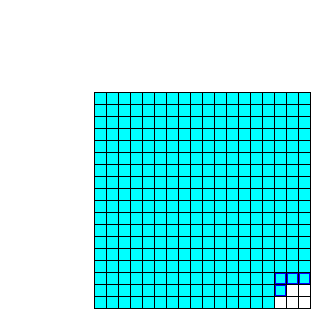The square root of 319 is 17.86057..., so 319 is just less than 18 squared. Thus, we see how much is left over when 319 is subtracted from 18 squared, which is 324, and we see that the answer is 5. Five is not itself a square number. It is one more than four, and it is, as shown by the squares outlined in blue in the diagram, four less than nine.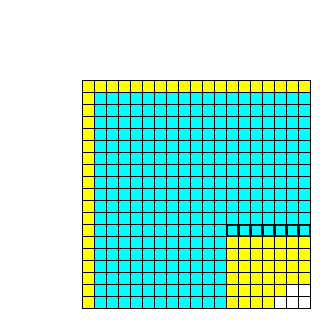Thus, we expand our square. We look at 19 squared. Thus, we expand our square on the outside by one row and one column, as shown by the yellow squares. This expands the overall square by 37 squares. The empty area on the inside grows by the same number of squares: so, the squares taken from the inside are also shown in yellow.Now, 19 squared minus 319 is 42, which is seven less than 49, as we see clearly after we finish expanding our test square.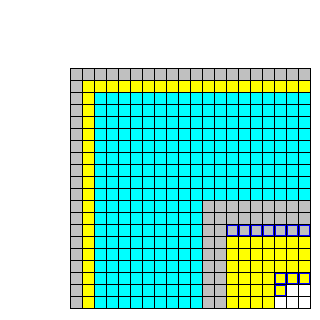This diagram shows the two changes we have made so far, and an additional one; now, we have moved to a 20 by 20 square, as shown by the 39 grey squares on the outside. Removing the same number of squares on the inside this time produces an empty space there of exactly 81 squares. So we have found our factors.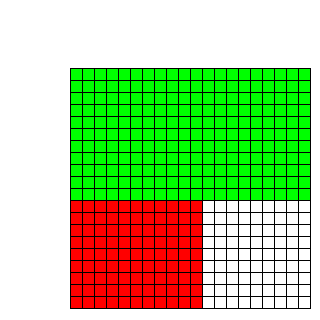This diagram shows why Fermat factorization works, once again. The difference of two squares produces an L-shaped region, and since the two arms of the L have the same thickness, the area shown in red can be rotated by 90 degrees and attached on the side of the green rectangle to form a longer rectangle.

Thus, in this example, since we have a 20x20 square with a 9x9 bite out of it, we have a 20x11 rectangle, and another 11x9 rectangle, and we can put them together to form a 29x11 rectangle, which shows that 319 is 29 times 11.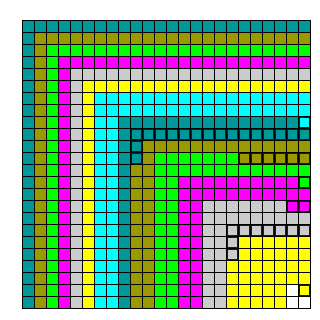Fermat factorization, however, only works well when the two factors of a number are close together. This diagram shows the first few steps involved in attempting to factor 321 using Fermat factorization. 321 is obviously 3 times 107, and 107 is a prime number, so there are no other factors.

Because the factors are so very different, Fermat factorization would continue until an L-shaped area only three squares thick, three being the smaller factor, is obtained, and that will be found in a 55x55 square.

Looking at this diagram, one notes that four consecutive regions of different colors in the interior of the square are about two rows thick. This suggests that it is possible to improve Fermat factorization, to allow one to skip several steps that one can tell will not be able to produce the final answer.

### Improving Fermat Factorization

Let us look at the steps shown in the diagram for factoring 321, to compare Fermat factorization with trial division.

```324 - 321 =   3 =   4 -  1    eliminating 16, 17, 18     (18 -  2 is 16)
361 - 321 =  40 =  49 -  9    eliminating 12, 13, 14, 15 (19 -  7 is 12)
400 - 321 =  79 =  81 -  2    eliminating 11             (20 -  9 is 11)
441 - 321 = 120 = 121 -  1    eliminating 10             (21 - 11 is 10)
484 - 321 = 163 = 169 -  6    eliminating  9             (22 - 13 is  9)
529 - 321 = 208 = 225 - 17    eliminating  8             (23 - 15 is  8)
576 - 321 = 255 = 256 -  1                               (24 - 16 is  8)
```

Thus we see that after the first few tries, Fermat factorization has, in this case, lost its advantage over trial division. However, that is because the regions involved are only two rows thick. For larger numbers, very many tries could still be required even in all but the very last stages, when Fermat factorization still retains its advantage over trial division.

As noted, four times in a row, the region being shifted outwards was about two rows thick on the inside. This can be used to speed up Fermat factorization because the differences between consecutive squares are the odd numbers. Thus, not only do successive L-shaped regions one row thick differ by two in the number of squares they contain, successive regions two rows thick differ by eight, successive regions three rows thick differ by eighteen, successive regions four rows thick differ by thirty-two, and so on (each difference being twice the square of the number of rows).

```Outside                   Inside                 Left Over
361 -> 400 (add 39)        49 ->  81 (add 32)    9 ->  2   (subtract  7)
```

What we see from this table gives us hope, but also shows that there is a problem. The number left over changes in a predictable pattern, but that pattern is not linear, it is quadratic.

### Extrapolation

How much can this speed up Fermat factorization? And what exactly are the steps involved? To try and see the answers to these questions, here is a more realistic example. In the book Codebreaker in the Far East, Alan Stripp, who worked as a British cryptanalyst during the Second World War, noted that his serial number in the Army was for a time 14429743, which his father informed him was the product of two prime numbers. How fitting that was, of course, would not be known for decades.

```[3799^2] 14432401 - 14429743 =  2658 =  [52^2]  2704 -  46 ; 3747 rows with  46 left
[3800^2] 14440000 - 14429743 = 10257 = [102^2] 10404 - 147 ; 3698 rows with 147 left
[3801^2] 14447601 - 14429743 = 17858 = [134^2] 17956 -  98 ; 3667 rows with  98 left
[3802^2] 14455204 - 14429743 = 25461 = [160^2] 25600 - 139 ; 3642 rows with 139 left
```

So far, the number of rows is changing quickly each time.

But this doesn't mean the optimization noted here is completely useless. The number of rows would change slowly if the inner square is near to the outer square in size. For a larger square, this would happen before the difference in sizes of the two squares involves only two rows, so the optimization would become useful while Fermat factorization remained more powerful than trial division.

Looking at the steps in Fermat factorization, however, long before the number of rows becomes constant over long stretches, it starts declining by exactly one for step after step. Hence:

```[4358^2] 18992164 - 14429743 = 4562421 = [2136^2] 4562496 -   75 ; 2222 rows with   75 left
[4359^2] 19000881 - 14429743 = 4571138 = [2139^2] 4575321 - 4183 ; 2220 rows with 4183 left
[4360^2] 19009600 - 14429743 = 4579857 = [2141^2] 4583881 - 4024 ; 2219 rows with 4024 left
[4361^2] 19018321 - 14429743 = 4588578 = [2143^2] 4592449 - 3871 ; 2218 rows with 3871 left
[4362^2] 19027044 - 14429743 = 4597301 = [2145^2] 4601025 - 3724 ; 2217 rows with 3724 left
...
[4412^2] 19465744 - 14429743 = 5036001 = [2245^2] 5040025 - 4024 ; 2167 rows with 4024 left
[4413^2] 19474569 - 14429743 = 5044826 = [2247^2] 5049009 - 4183 ; 2166 rows with 4183 left
[4414^2] 19483396 - 14429743 = 5053653 = [2249^2] 5058001 - 4348 ; 2165 rows with 4348 left
[4415^2] 19492225 - 14429743 = 5062482 = [2250^2] 5062500 -   18 ; 2165 rows with   18 left
```

Since the number of rows is changing at a constant rate, this can be handled by a formula as well.

The big square starts at 4359 squares on a side, at the beginning of the uniform stretch. Thus, it grows by 8719 squares, then 8721 squares, then 8723 squares, and so on, two more each time.

The little square starts at 2139 squares on a side, and grows by two rows each time. So it grows by 8560 squares, then 8568 squares, then 8576 squares, and so on, eight more each time.

The number of odd squares left over first changes from 4183 to 4024, for a decrease of 159 squares. Each succeeding time, this decrease itself decreases (eventually changing to an increase) by six squares each time.

Thus, one has a quadratic equation for the number of squares left over. How do we use it to find out if that number becomes zero, and to find out when the uniform stretch ends, and a new calculation is needed?

Finding where a quadratic equation yields zero is, of course, easy enough.

```   2
a x + b x + c = 0
```

when

```            ________
/  2
-b ± \/  b - 4ac
x = ----------------
2a
```

as we learned in school.

The stretch ends when the number left over either becomes negative, or exceeds the size of the outermost row of the inner empty square; the former case is indicated by the roots of the quadratic, and since the second case involves the intersection of the quadratic with a straight line, subtracting the equation from the straight line from that of the quadratic yields another quadratic.

This is but the beginning of the ways in which Fermat factorization may be accelerated by extrapolation.

### Enlargement

Given that Fermat factorization works best when the factors are equal in size, if the two factors, p and q, of the number being factored happen to be such that p is about three times as large as q, then trying to factor 3pq would first turn up p and 3q as the factors.

Thus, one could first try to factor pq. When the L-shaped region has a large enough bite out of it as to indicate factorizations into two components differing by a factor of three have been reached, one could switch to trying to factor 3pq. Then, when the two components differ by a ration of 5/3, switch to trying to factor 5pq, and so on.

Where the little square is of side b, and the big square of side a, one is comparing the ratio between a+b and a-b, since those are the candidates for p and q, to determine what ratio between p and q has been reached.

Since a+b and a-b differ by 2b, we have been only considering the case where p and q are both odd. But this would also work if they were both even, so one could also, for example, try to factor 8pq; if p were about twice the size of q, twice p and four times q would turn up early in the search for factors.

### The Factor Base Algorithm

If we are now looking for cases where a squared minus b squared equals some multiple of pq, why not simply look for cases where a squared and b squared are equal modulo pq?

Not all such cases, though, will really factor pq. After all, 107pq can be factored into 107 and pq.

The factor base method works like this: starting with the numbers just after the square root of pq, the number to be factored, find those numbers which are the product only of numbers from a set of small prime numbers.

Once one has enough such numbers, one can find among them a set of numbers which, when multiplied together, produces a result with only even powers of those prime numbers. The two products of any two pieces in which you split that set will be the kind of number you are looking for. (In practice, the number -1 is included in addition to several small prime numbers; this doubles the chance of dealing with a smaller number, which is more likely to be the product of small primes.)

Instead of looking at all the numbers after the square root of pq, numbers likely to be small modulo pq can be found by the continued fraction method of factoring. A more complicated method of even more quickly finding numbers worth trying, which requires a special choice of the small prime numbers, is called the quadratic sieve method. Even more advanced and efficient methods of factoring now exist.

[Next] [Up] [Previous] [Index]